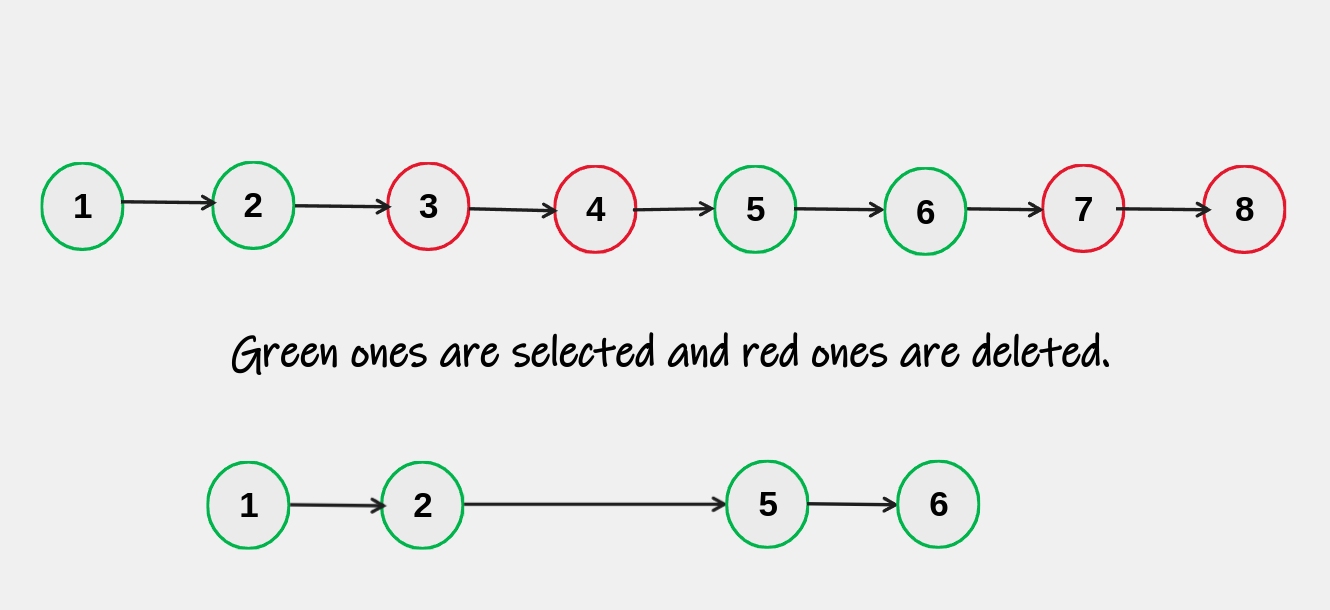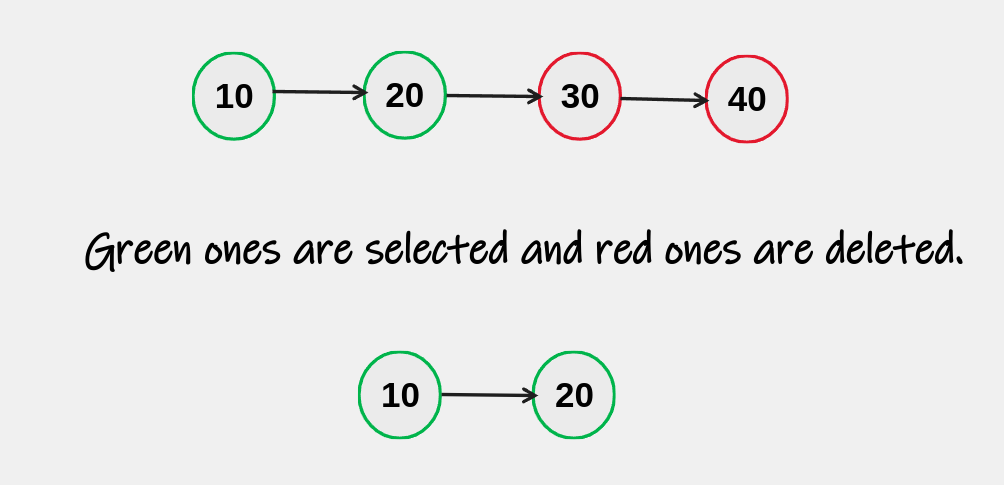New update is available. Click here to update.

# Delete Nodes On Regular Intervals

Contributed by
Prashant Thakur
Last Updated: 23 Feb, 2023
Ninja0/200
Avg time to solve 20 mins
Success Rate 67 %Share0 upvotes

## Problem Statement

#### You are given two integers, ‘A’ and ‘B’, and the ‘head’ of a linked list. You are also given ‘N’, denoting the number of nodes in the linked list. You have to return the head of the modified, linked list by removing the nodes in the following way:

• Starting with the current node, keep the first ‘A’ nodes.
• Remove the next ‘B’ nodes.
• Repeat steps 2 and 3 until we have reached the end of the node.
##### Example:
``````Input: ‘A’ = 2, ‘B’ = 2, ‘N’ = 8, ‘head’ = [1, 2, 3, 4, 5, 6, 7, 8]

Output: [1, 2, 5, 6]

Explanation: Keep the first A(2) nodes, delete the next B(2) nodes, and continue until we have reached the end of the linked list.
``````
Detailed explanation ( Input/output format, Notes, Images )##### Constraints:
``````1 <= T <= 10
1 <= N <= 1e3
1 <= A, B <= 100
The value of each node in the linked list is in the range [1 to 1e9]

Time Limit: 1-sec
``````
##### Sample Input 1:
``````2
2 2
8
1 2 3 4 5 6 7 8

2 3
4
10 20 30 40
``````
##### Sample Output 1:
``````1 2 5 6
10 20
``````
##### Explanation Of Sample Input 1:
``````For test case 1:

Input: ‘A’ = 2, ‘B’ = 2, ‘N’ = 8, ‘head’ = [1, 2, 3, 4, 5, 6, 7, 8]

Output: [1, 2, 5, 6]

Explanation: Keep the first A(2) nodes, delete the next B(2) nodes, and continue until we have reached the end of the linked list.
````````````For test case 2:

Input: ‘A’ = 2, ‘B’ = 2, ‘N’ = 8, ‘head’ = [1, 2, 3, 4, 5, 6, 7, 8]

Output: [1, 2, 5, 6]

Explanation: Keep the first A(2) nodes, delete the next B(3) nodes, and continue until we have reached the end of the linked list.
``````##### Sample Input 2:
``````2
10 10
1
8

1 1
4
9 8 7 6
``````
##### Sample Output 2:
``````8
9 7
``````AutoConsole